• ## EXCEL函数公式集

热门讨论 2010-03-16 03:26:38
excel常用函数公式及技巧搜集 从身份证号码中提取出生年月日 从身份证号码中提取出性别 从身份证号码中进行年龄判断 按身份证号号码计算至今天年龄 以2006年10月31日为基准日,按按身份证计算年龄(周岁)公式 按...
• 1、把公式产生错误显示为空 2、IF多条件判断返回值 三、统计公式 1、统计两个表格重复的内容 2、统计不重复的总人数 四、求和公式 1、隔列求和 2、单条件求和 3、单条件模糊求和 4、多条件模糊求和 5、多表相同...


一、数字处理
1、取绝对值
2、取整
3、四舍五入
二、判断公式
1、把公式产生的错误值显示为空
2、IF多条件判断返回值
三、统计公式
1、统计两个表格重复的内容
2、统计不重复的总人数
四、求和公式
1、隔列求和
2、单条件求和
3、单条件模糊求和
4、多条件模糊求和
5、多表相同位置求和
6、按日期和产品求和
五、查找与引用公式
1、单条件查找公式
2、双向查找公式
3、查找最后一条符合条件的记录。
4、多条件查找
5、指定区域最后一个非空值查找
6、按数字区域间取对应的值
六、字符串处理公式
1、多单元格字符串合并
2、截取除后3位之外的部分
3、截取-前的部分
4、截取字符串中任一段的公式
5、字符串查找
6、字符串查找一对多
七、日期计算公式
1、两日期相隔的年、月、天数计算
2、扣除周末天数的工作日天数

一、数字处理

1、取绝对值

=ABS(数字)

2、取整

=INT(数字)

3、四舍五入

=ROUND(数字,小数位数)

二、判断公式

1、把公式产生的错误值显示为空

公式：C2
=IFERROR(A2/B2,"")

说明：如果是错误值则显示为空，否则正常显示。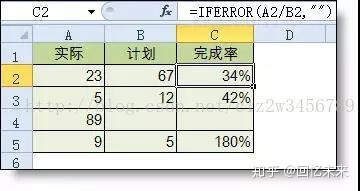2、IF多条件判断返回值
公式：C2
=IF(AND(A2<500,B2="未到期"),"补款","")

说明：两个条件同时成立用AND,任一个成立用OR函数。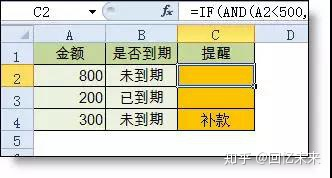三、统计公式

1、统计两个表格重复的内容

公式:B2
=COUNTIF(Sheet15!A:A,A2)

说明：如果返回值大于0说明在另一个表中存在，0则不存在。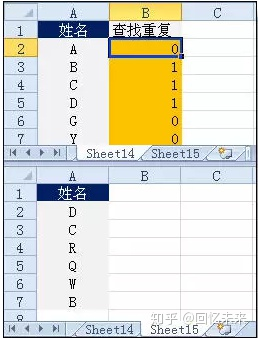2、统计不重复的总人数

公式：C2

=SUMPRODUCT(1/COUNTIF(A2:A8,A2:A8))

说明:用COUNTIF统计出每人的出现次数，用1除的方式把出现次数变成分母，然后相加。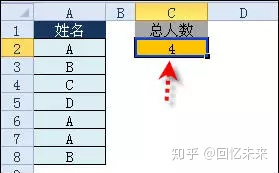四、求和公式

1、隔列求和
公式：H3

=SUMIF($A$2:$G$2,H$2,A3:G3) 或 =SUMPRODUCT((MOD(COLUMN(B3:G3),2)=0)*B3:G3) 说明：如果标题行没有规则用第2个公式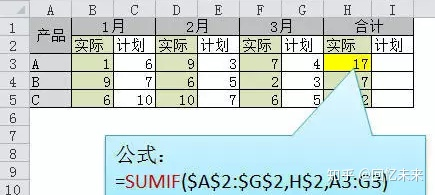2、单条件求和 公式：F2 =SUMIF(A:A,E2,C:C) 说明：SUMIF函数的基本用法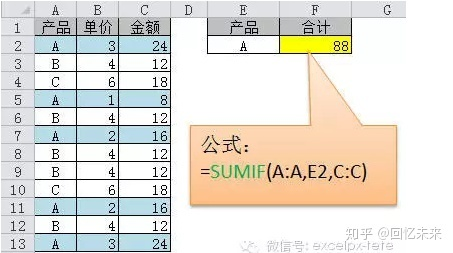3、单条件模糊求和 公式：详见下图 说明：如果需要进行模糊求和，就需要掌握通配符的使用，其中星号是表示任意多个字符，如"*A*"就表示a前和后有任意多个字符，即包含A。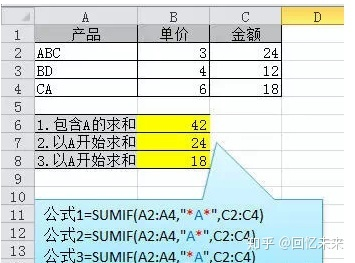4、多条件模糊求和 公式：C11 =SUMIFS(C2:C7,A2:A7,A11&"*",B2:B7,B11) 说明：在sumifs中可以使用通配符*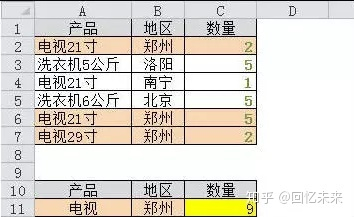5、多表相同位置求和 公式：b2 =SUM(Sheet1:Sheet19!B2) 说明：在表中间删除或添加表后，公式结果会自动更新。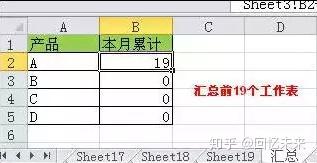6、按日期和产品求和 公式：F2 =SUMPRODUCT((MONTH($A$2:$A$25)=F$1)*($B$2:$B$25=$E2)*$C$2:$C$25) 说明：SUMPRODUCT可以完成多条件求和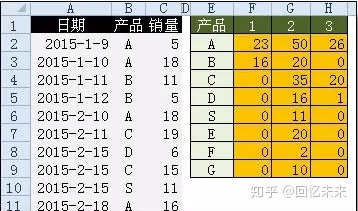五、查找与引用公式 1、单条件查找公式 公式1：C11 =VLOOKUP(B11,B3:F7,4,FALSE) 说明：查找是VLOOKUP最擅长的，基本用法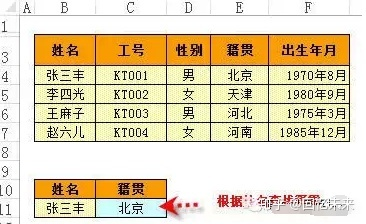2、双向查找公式 公式： =INDEX(C3:H7,MATCH(B10,B3:B7,0),MATCH(C10,C2:H2,0)) 说明：利用MATCH函数查找位置，用INDEX函数取值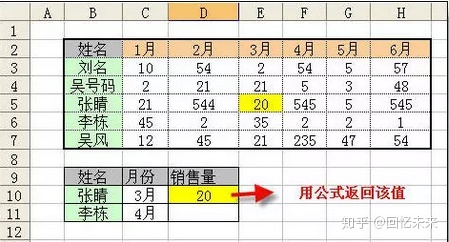3、查找最后一条符合条件的记录。 公式：详见下图 说明：0/(条件)可以把不符合条件的变成错误值，而lookup可以忽略错误值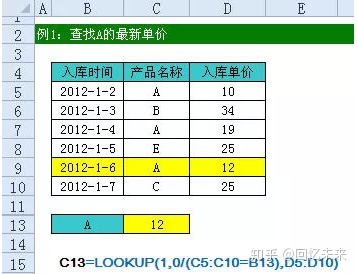4、多条件查找 公式:详见下图 说明:公式原理同上一个公式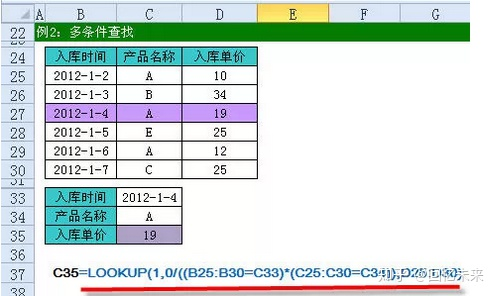5、指定区域最后一个非空值查找 公式;详见下图 说明：略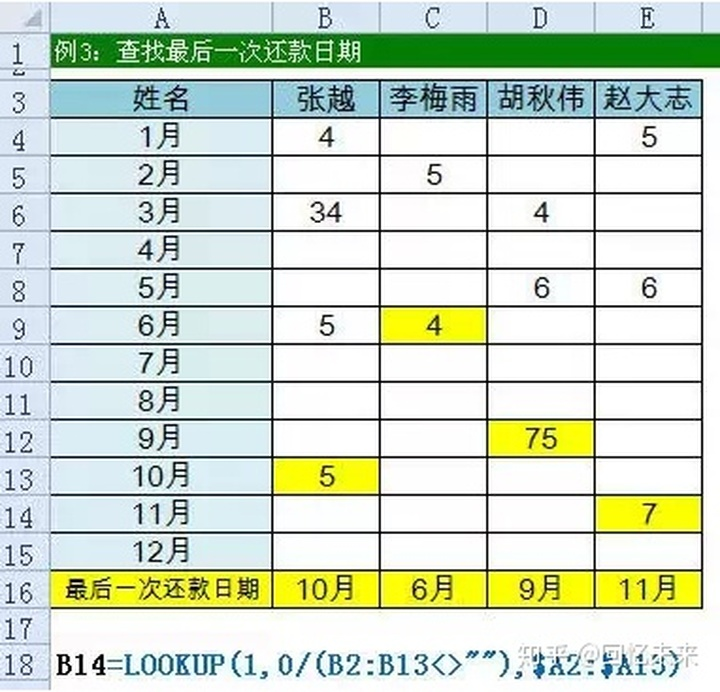6、按数字区域间取对应的值 公式：详见下图 公式说明：VLOOKUP和LOOKUP函数都可以按区间取值，一定要注意，销售量列的数字一定要升序排列。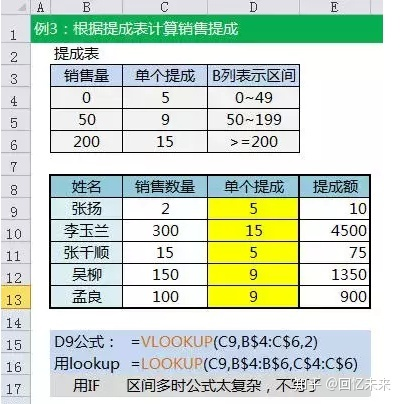六、字符串处理公式 1、多单元格字符串合并 公式：c2 =PHONETIC(A2:A7) 说明：Phonetic函数只能对字符型内容合并，数字不可以。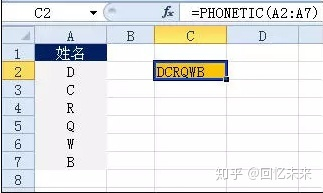2、截取除后3位之外的部分 公式： =LEFT(D1,LEN(D1)-3) 说明：LEN计算出总长度,LEFT从左边截总长度-3个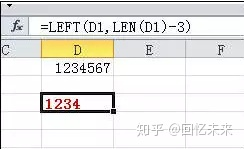3、截取-前的部分 公式:B2 =Left(A1,FIND("-",A1)-1) 说明：用FIND函数查找位置，用LEFT截取。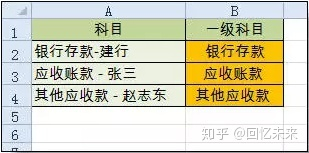4、截取字符串中任一段的公式 公式:B1 =TRIM(MID(SUBSTITUTE($A1," ",REPT(" ",20)),20,20))

说明:公式是利用强插N个空字符的方式进行截取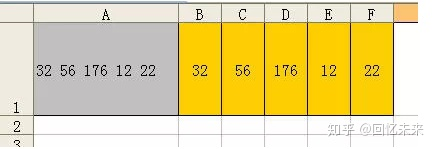5、字符串查找

公式：B2
=IF(COUNT(FIND("河南",A2))=0,"否","是")

说明: FIND查找成功，返回字符的位置，否则返回错误值，而COUNT可以统计出数字的个数，这里可以用来判断查找是否成功。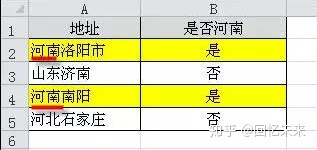6、字符串查找一对多
公式：B2
=IF(COUNT(FIND({"辽宁","黑龙江","吉林"},A2))=0,"其他","东北")

说明：设置FIND第一个参数为常量数组，用COUNT函数统计FIND查找结果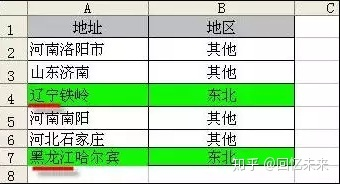七、日期计算公式

1、两日期相隔的年、月、天数计算

A1是开始日期（2011-12-1），B1是结束日期(2013-6-10)。计算：
相隔多少天？=datedif(A1,B1,"d") 结果：557

相隔多少月? =datedif(A1,B1,"m") 结果：18

相隔多少年? =datedif(A1,B1,"Y") 结果：1

不考虑年相隔多少月？=datedif(A1,B1,"Ym") 结果：6

不考虑年相隔多少天？=datedif(A1,B1,"YD") 结果：192

不考虑年月相隔多少天？=datedif(A1,B1,"MD") 结果：9

datedif函数第3个参数说明：

"Y" 时间段中的整年数。

"M" 时间段中的整月数。

"D" 时间段中的天数。

"MD" 天数的差。忽略日期中的月和年。

"YM" 月数的差。忽略日期中的日和年。

"YD" 天数的差。忽略日期中的年。

2、扣除周末天数的工作日天数

公式：C2

=NETWORKDAYS.INTL(IF(B2<DATE(2015,1,1),DATE(2015,1,1),B2),DATE(2015,1,31),11)

说明：返回两个日期之间的所有工作日数，使用参数指示哪些天是周末，以及有多少天是周末。周末和任何指定为假期的日期不被视为工作日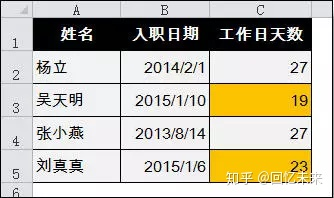展开全文excel
• @DbLookup 1 给定一个值，在一个指定的视图（或文件夹）里查找，找出视图里的第一个排序列里包含该值的所有文档。对每个选中的文档，@DbLookup 返回视图里指定列的内容，或者返回一个指定域的内容 2 使用 ODBC.INI ...
• (1)确定列表中某个值的位置； (2)对某个输入值进行检验，确定这个值是否存在某个列表中； (3)判断某列表中是否存在重复数据； (4)定位某一列表中最后一个非空单元格的位置。 查找文本值时，函数 MATCH 不区分大小写...
简介
今天要介绍的是，根据查找单元格内容来进行类别匹配，如图所示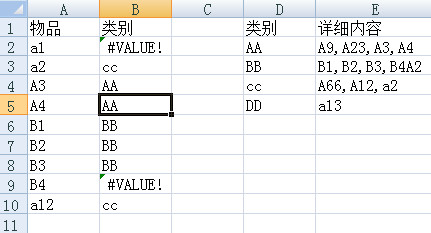MATCH函数
简介
匹配函数,指返回指定数值在指定数组区域中的位置。
用途：
(1)确定列表中某个值的位置；
(2)对某个输入值进行检验，确定这个值是否存在某个列表中；
(3)判断某列表中是否存在重复数据；
(4)定位某一列表中最后一个非空单元格的位置。
查找文本值时，函数 MATCH 不区分大小写字母。
语法：
MATCH(lookup_value, lookup_array, match_type)

参数
简单说明
数据类型
备注

lookup_value
需要在数据表（lookup_array）中查找的值
数值，文本，单元格引用
可以包含通配符，*和？，星号可以匹配任何字符序列；问号可以匹配单个字符

lookup_array
可能包含有所要查找数值的连续的单元格区域
一维数组
区域必须是某一行或某一列

match_type
表示查询的指定方式
-1、0或1，默认为1

1：查找小于或等于lookup_value的最大数值在lookup_array中的位置，lookup_array必须按升序排列：否则，当遇到比lookup_value更大的值时，即时终止查找并返回此值之前小于或等于lookup_value的数值的位置。如果lookup_array的值均小于或等于lookup_value，则返回数组最后一个值的位置；如果lookup_array的值均大于lookup_value，则返回#N/A。

0：查找等于lookup_value的第一个数值，lookup_array按任意顺序排列；

-1：查找大于或等于lookup_value的最小数值在lookup_array中的位置，lookup_array必须按降序排列；否则，当遇到比lookup_value更小的值时，即时终止查找并返回此值之前大于或等于lookup_value的数值的位置。如果lookup_array的值均大于或等于lookup_value，则返回数组最后一个值的位置；如果lookup_array的值均小于lookup_value，则返回#N/A。

使用：
查找E列，内容包含A2单元格的行号
=MATCH("*"&A2&"*",$E:$E,0)

逗号匹配
假设单元格内容以逗号区别项目，为了使相似值不互相影响（类似str和string），因此要进行区别匹配
//only 只有一个项目，例如单元格只有str一个字符
=IF(ISERROR(MATCH(A2,$E:$E,0)),"无",MATCH(A2,$E:$E,0))

//begin 项目在第一个位置，例如单元格内容为str，abc
=IF(ISERROR(MATCH(A2&",*",$E:$E,0)),"无",MATCH(A2&",*",$E:$E,0))

//mid 项目在中间位置，例如单元格内容为a，str，b
=IF(ISERROR(MATCH("*,"&A2&",*",$E:$E,0)),"无",MATCH("*,"&A2&",*",$E:$E,0))

//end 项目在末尾，例如单元格内容为a,str
=IF(ISERROR(MATCH("*,"&A2,$E:$E,0)),"无",MATCH("*,"&A2,$E:$E,0))

完整代码
通过几个if语句判断返回查找内容的行号，ADDRESS(行号，列号)得到查找到的对应的类别地址，INDIRECT（地址）获取此地址的值
=INDIRECT(ADDRESS(IF(ISERROR(MATCH(A3&",*",$E:$E,0)),IF(ISERROR(MATCH("*,"&A3&",*",$E:$E,0)),IF(ISERROR(MATCH("*,"&A3,$E:$E,0)),IF(ISERROR(MATCH(A3,$E:$E,0)),"无",MATCH(A3,$E:$E,0)),MATCH("*,"&A3,$E:$E,0)),MATCH("*,"&A3&",*",$E:$E,0)),MATCH(A3&",*",$E:$E,0)),CELL("col",$E1)-1))   展开全文MATCH • 本书既可以作为函数速查工具手册，又可以作为丰富的函数应用案例宝典，适合对Excel 公式与函数有需求的读者阅读。 第1章 公式与函数基础 1 1.1 了解公式 1 1.1.1 公式的组成部分 1 1.1.2 数据类型及其相互转换 2... • 8.4.2 查找值的左侧 8.4.3 进行区分大小写的查找 8.4.4 在多个查找表中进行选择 8.4.5 确定考试分数的等级 8.4.6 计算等级平均分（GPA） 8.4.7 进行双向查找 8.4.8 进行双列查找 8.4.9 确定范围中值的... • 8.4.2 查找值的左侧 8.4.3 进行区分大小写的查找 8.4.4 在多个查找表中进行选择 8.4.5 确定考试分数的等级 8.4.6 计算等级平均分（GPA） 8.4.7 进行双向查找 8.4.8 进行双列查找 8.4.9 确定范围中值的... • 查找重复值 公式：=IF(COUNTIF(A:A,A2)&gt;1,“重复”,"") 选定单元格，然后输入函数公式：=IF(COUNTIF(A:A,A2)&gt;1,“重复”,"")，当光标变成"+"时下拉即可。 2.成绩排名... Excel函数是一个比较难以攻克的难题，很多朋友都想学，但又不知道该样学，今天小编专门为大家整理6个容易上手的Excel函数小技巧，想学的赶紧来学。查找重复值 公式：=IF(COUNTIF(A:A,A2)>1,“重复”,"") 选定单元格，然后输入函数公式：=IF(COUNTIF(A:A,A2)>1,“重复”,"")，当光标变成"+"时下拉即可。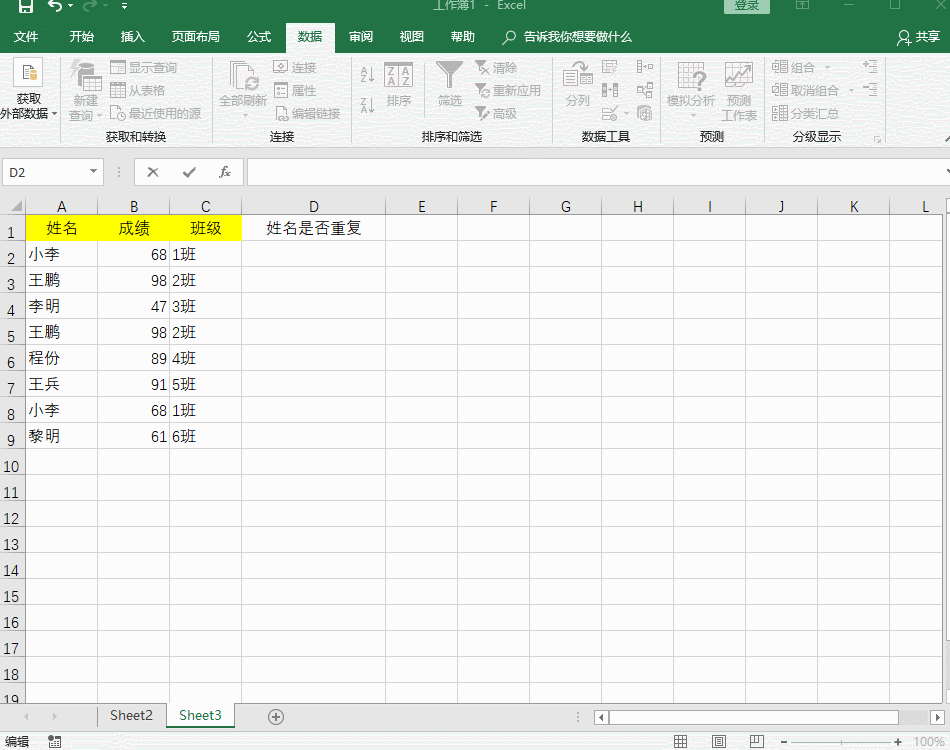2.成绩排名 公式：=RANK.EQ(B2,B$2:B$9) 在众多的数据中，算出成绩排名，在选定的单元格中输入函数公式：=RANK.EQ(B2,B$2:B\$9)，当光标变成"+"时下拉即可，从而获得排名数据，是不是很方便快捷呀？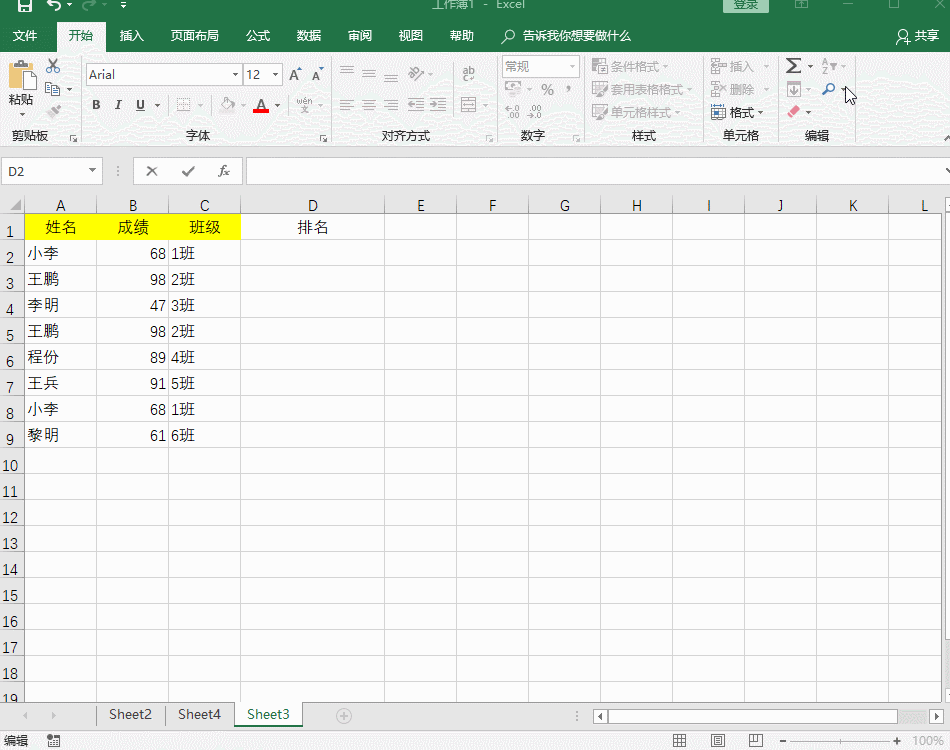合并多个单元格内容

公式：=A2&B2&C2&D2
将多个单元格内容合并到一个单元格中，重要利用【&】符号使得单元格串联起来，，就可以实现合并多个单元格内容。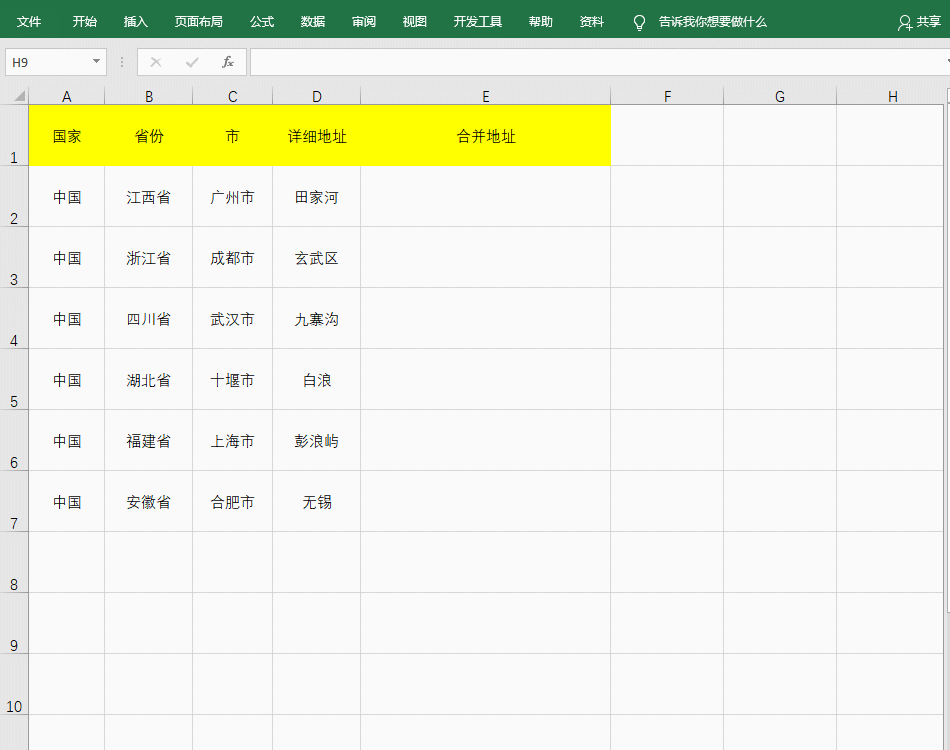4.一键求和公式
公式：=SUM(B2:D2)
在计算总数的单元格中输入函数公式：=SUM(B2:D2),然后下拉即可实现一键求和。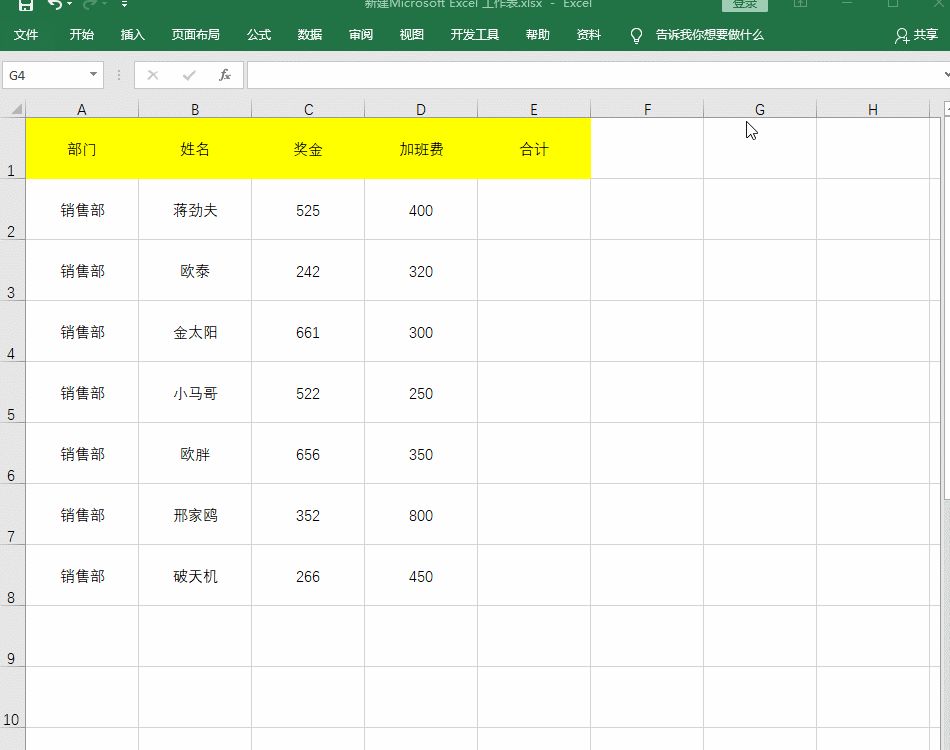5.随机生成字母
公式：=CHAR(65+RAND()*26)
随机选定单元格，然后输入函数公式：=CHAR(65+RAND()*26)，然后下拉即可生成字母。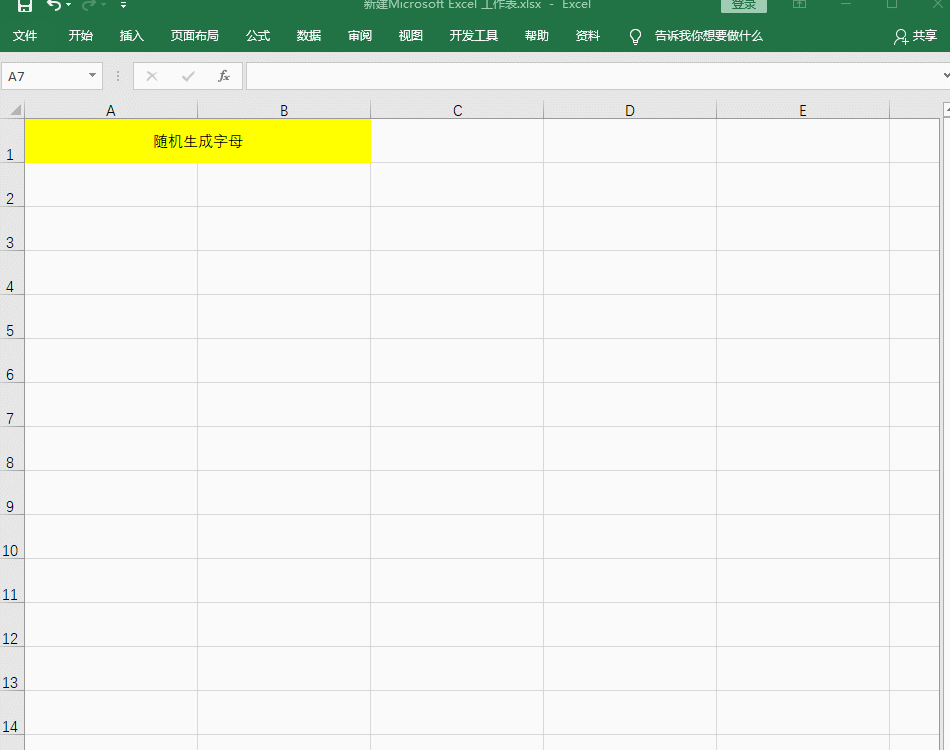判断表格数据

公式：=IF(B2>60,“及格”,“不及格”)
判断表格中成绩合不合格，选定单元格，然后输入公式：=IF(B2>60,“及格”,“不及格”)，下拉即可。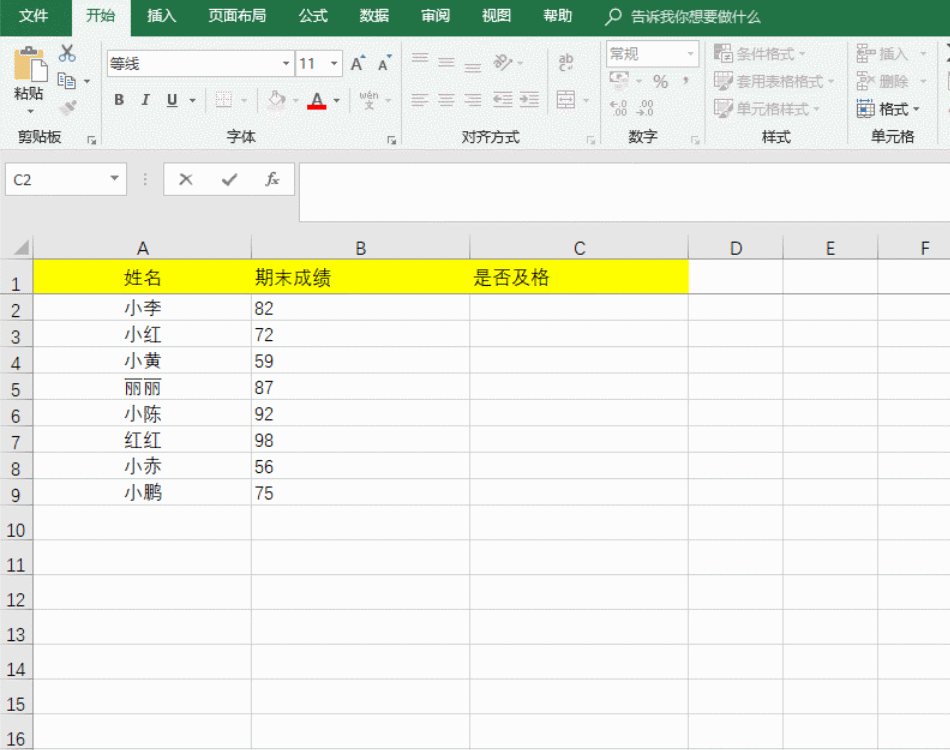好了，今天的Excel函数分享就要结束了，有需要的赶紧拿走哦！


展开全文• ## 三角函数计算，Cordic 算法入门

万次阅读 多人点赞 2013-01-02 13:47:45
这种表格在人们刚刚产生三角函数的概念时候就已经有了，它们通常是通过从已知（比如sin(π/2)=1）开始并重复应用半角和和差公式而生成。 现在有了计算机，三角函数表便推出了历史舞台。但是像我这样喜欢...
三角函数计算，Cordic 算法入门

三角函数的计算是个复杂的主题，有计算机之前，人们通常通过查找三角函数表来计算任意角度的三角函数的值。这种表格在人们刚刚产生三角函数的概念的时候就已经有了，它们通常是通过从已知值（比如sin(π/2)=1）开始并重复应用半角和和差公式而生成。

现在有了计算机，三角函数表便推出了历史的舞台。但是像我这样的喜欢刨根问底的人，不禁要问计算机又是如何计算三角函数值的呢。最容易想到的办法就是利用级数展开，比如泰勒级数来逼近三角函数，只要项数取得足够多就能以任意的精度来逼近函数值。除了泰勒级数逼近之外，还有其他许多的逼近方法，比如切比雪夫逼近、最佳一致逼近和Padé逼近等。
所有这些逼近方法本质上都是用多项式函数来近似我们要计算的三角函数，计算过程中必然要涉及到大量的浮点运算。在缺乏硬件乘法器的简单设备上（比如没有浮点运算单元的单片机），用这些方法来计算三角函数会非常的费时。为了解决这个问题，J. Volder于1959年提出了一种快速算法，称之为CORDIC(COordinate Rotation DIgital Computer) 算法，这个算法只利用移位和加减运算，就能计算常用三角函数值，如Sin，Cos，Sinh，Cosh等函数。 J. Walther在1974年在这种算法的基础上进一步改进，使其可以计算出多种超越函数，更大的扩展了Cordic 算法的应用。因为Cordic 算法只用了移位和加法，很容易用纯硬件来实现，因此我们常能在FPGA运算平台上见到它的身影。不过，大多数的软件程序员们都没有听说过这种算法，也更不会主动的去用这种算法。其实，在嵌入式软件开发，尤其是在没有浮点运算指令的嵌入式平台（比如定点型DSP）上做开发时，还是会遇上可以用到Cordic 算法的情况的，所以掌握基本的Cordic算法还是有用的。

从二分查找法说起

先从一个例子说起，知道平面上一点在直角坐标系下的坐标（X,Y）=（100，200），如何求的在极坐标系下的坐标（ρ,θ）。用计算器计算一下可知答案是（223.61，63.435）。图 1 直角坐标系到极坐标系的转换
为了突出重点，这里我们只讨论X和Y都为正数的情况。这时θ=atan(y/x)。求θ的过程也就是求atan 函数的过程。Cordic算法采用的想法很直接，将（X，Y）旋转一定的度数，如果旋转完纵坐标变为了0，那么旋转的度数就是θ。坐标旋转的公式可能大家都忘了，这里把公式列出了。设（x,y）是原始坐标点，将其以原点为中心，顺时针旋转θ之后的坐标记为（x’,y’）,则有如下公式：也可以写为矩阵形式：如何旋转呢，可以借鉴二分查找法的思想。我们知道θ的范围是0到90度。那么就先旋转45度试试。旋转之后纵坐标为70.71，还是大于0，说明旋转的度数不够，接着再旋转22.5度（45度的一半）。这时总共旋转了45+22.5=67.5度。结果纵坐标变为了负数，说明θ<67.5度，这时就要往回转，还是二分查找法的思想，这次转11.25度。这时总共旋转了45+22.5-11.25=56.25度。又转过头了，接着旋转，这次顺时针转5.625度。这时总共旋转了45+22.5-11.25+5.625=61.875度。这时纵坐标已经很接近0了。我们只是说明算法的思想，因此就不接着往下计算了。计算到这里我们给的答案是 61.875±5.625。二分查找法本质上查找的是一个区间，因此我们给出的是θ值的一个范围。同时，坐标到原点的距离ρ也求出来了，ρ=223.52。与标准答案比较一下计算的结果还是可以的。旋转的过程图示如下。可能有读者会问，计算中用到了 sin 函数和 cos 函数，这些值又是怎么计算呢。很简单，我们只用到很少的几个特殊点的sin 函数和 cos 函数的值，提前计算好存起来，用时查表。
下面给出上面方法的C 语言实现。
#include <stdio.h>
#include <stdlib.h>

double my_atan2(double x, double y);
int main(void)
{
double z = my_atan2(100.0, 200.0);
printf("\n z = %f \n", z);

return 0;
}

double my_atan2(double x, double y)
{
const double sine[] = {0.7071067811865,0.3826834323651,0.1950903220161,0.09801714032956,
0.04906767432742,0.02454122852291,0.01227153828572,0.006135884649154,0.003067956762966
,0.001533980186285,7.669903187427045e-4,3.834951875713956e-4,1.917475973107033e-4,
9.587379909597735e-5,4.793689960306688e-5,2.396844980841822e-5
};

const double cosine[] = {0.7071067811865,0.9238795325113,0.9807852804032,0.9951847266722,
0.9987954562052,0.9996988186962,0.9999247018391,0.9999811752826,0.9999952938096,
0.9999988234517,0.9999997058629,0.9999999264657,0.9999999816164,0.9999999954041,
0.999999998851,0.9999999997128
};

int i = 0;
double x_new, y_new;
double angleSum = 0.0;
double angle = 45.0;

for(i = 0; i < 15; i++)
{
if(y > 0)
{
x_new = x * cosine[i] + y * sine[i];
y_new = y * cosine[i] - x * sine[i];
x = x_new;
y = y_new;
angleSum += angle;
}
else
{
x_new = x * cosine[i] - y * sine[i];
y_new = y * cosine[i] + x * sine[i];
x = x_new;
y = y_new;
angleSum -= angle;
}
printf("Debug: i = %d angleSum = %f, angle = %f\n", i, angleSum, angle);
angle /= 2;
}
return angleSum;
}程序运行的输出结果如下：
Debug: i = 0 angleSum = 45.000000, angle = 45.000000
Debug: i = 1 angleSum = 67.500000, angle = 22.500000
Debug: i = 2 angleSum = 56.250000, angle = 11.250000
Debug: i = 3 angleSum = 61.875000, angle = 5.625000
Debug: i = 4 angleSum = 64.687500, angle = 2.812500
Debug: i = 5 angleSum = 63.281250, angle = 1.406250
Debug: i = 6 angleSum = 63.984375, angle = 0.703125
Debug: i = 7 angleSum = 63.632813, angle = 0.351563
Debug: i = 8 angleSum = 63.457031, angle = 0.175781
Debug: i = 9 angleSum = 63.369141, angle = 0.087891
Debug: i = 10 angleSum = 63.413086, angle = 0.043945
Debug: i = 11 angleSum = 63.435059, angle = 0.021973
Debug: i = 12 angleSum = 63.424072, angle = 0.010986
Debug: i = 13 angleSum = 63.429565, angle = 0.005493
Debug: i = 14 angleSum = 63.432312, angle = 0.002747

z = 63.432312减少乘法运算
现在已经有点 Cordic 算法的样子了，但是我们看到没次循环都要计算 4 次浮点数的乘法运算，运算量还是太大了。还需要进一步的改进。改进的切入点当然还是坐标变换的过程。我们将坐标变换公式变一下形。可以看出 cos(θ)可以从矩阵运算中提出来。我们可以做的再彻底些，直接把 cos(θ) 给省略掉。省略cos(θ)后发生了什么呢，每次旋转后的新坐标点到原点的距离都变长了，放缩的系数是1/cos(θ)。不过没有关系，我们求的是θ，不关心ρ的改变。这样的变形非常的简单，但是每次循环的运算量一下就从4次乘法降到了2次乘法了。
还是给出 C 语言的实现：
double my_atan3(double x, double y)
{
const double tangent[] = {1.0,0.4142135623731,0.1989123673797,0.09849140335716,0.04912684976947,
0.02454862210893,0.01227246237957,0.006136000157623,0.003067971201423,
0.001533981991089,7.669905443430926e-4,3.83495215771441e-4,1.917476008357089e-4,
9.587379953660303e-5,4.79368996581451e-5,2.3968449815303e-5
};

int i = 0;
double x_new, y_new;
double angleSum = 0.0;
double angle = 45.0;

for(i = 0; i < 15; i++)
{
if(y > 0)
{
x_new = x + y * tangent[i];
y_new = y - x * tangent[i];
x = x_new;
y = y_new;
angleSum += angle;
}
else
{
x_new = x - y * tangent[i];
y_new = y + x * tangent[i];
x = x_new;
y = y_new;
angleSum -= angle;
}
printf("Debug: i = %d angleSum = %f, angle = %f, ρ = %f\n", i, angleSum, angle, hypot(x,y));
angle /= 2;
}
return angleSum;
}计算的结果是：
Debug: i = 0 angleSum = 45.000000, angle = 45.000000, ρ = 316.227766
Debug: i = 1 angleSum = 67.500000, angle = 22.500000, ρ = 342.282467
Debug: i = 2 angleSum = 56.250000, angle = 11.250000, ρ = 348.988177
Debug: i = 3 angleSum = 61.875000, angle = 5.625000, ρ = 350.676782
Debug: i = 4 angleSum = 64.687500, angle = 2.812500, ρ = 351.099697
Debug: i = 5 angleSum = 63.281250, angle = 1.406250, ρ = 351.205473
Debug: i = 6 angleSum = 63.984375, angle = 0.703125, ρ = 351.231921
Debug: i = 7 angleSum = 63.632813, angle = 0.351563, ρ = 351.238533
Debug: i = 8 angleSum = 63.457031, angle = 0.175781, ρ = 351.240186
Debug: i = 9 angleSum = 63.369141, angle = 0.087891, ρ = 351.240599
Debug: i = 10 angleSum = 63.413086, angle = 0.043945, ρ = 351.240702
Debug: i = 11 angleSum = 63.435059, angle = 0.021973, ρ = 351.240728
Debug: i = 12 angleSum = 63.424072, angle = 0.010986, ρ = 351.240734
Debug: i = 13 angleSum = 63.429565, angle = 0.005493, ρ = 351.240736
Debug: i = 14 angleSum = 63.432312, angle = 0.002747, ρ = 351.240736

z = 63.432312消除乘法运算
我们已经成功的将乘法的次数减少了一半，还有没有可能进一步降低运算量呢？还要从计算式入手。第一次循环时，tan(45)=1，所以第一次循环实际上是不需要乘法运算的。第二次运算呢？
Tan(22.5)=0.4142135623731,很不幸，第二次循环乘数是个很不整的小数。是否能对其改造一下呢？答案是肯定的。第二次选择22.5度是因为二分查找法的查找效率最高。如果选用个在22.5到45度之间的值，查找的效率会降低一些。如果稍微降低一点查找的效率能让我们有效的减少乘法的次数，使最终的计算速度提高了，那么这种改进就是值得的。
我们发现tan(26.565051177078)=0.5，如果我们第二次旋转采用26.565051177078度，那么乘数变为0.5，如果我们采用定点数运算的话（没有浮点协处理器时为了加速计算我们会大量的采用定点数算法）乘以0.5就相当于将乘数右移一位。右移运算是很快的，这样第二次循环中的乘法运算也被消除了。
类似的方法，第三次循环中不用11.25度，而采用 14.0362434679265 度。
Tan(14.0362434679265)= 1/4
乘数右移两位就可以了。剩下的都以此类推。
tan(45)= 1
tan(26.565051177078)= 1/2
tan(14.0362434679265)= 1/4
tan(7.1250163489018)= 1/8
tan(3.57633437499735)= 1/16
tan(1.78991060824607)= 1/32
tan(0.8951737102111)= 1/64
tan(0.4476141708606)= 1/128
tan(0.2238105003685)= 1/256还是给出C语言的实现代码，我们采用循序渐进的方法，先给出浮点数的实现（因为用到了浮点数，所以并没有减少乘法运算量，查找的效率也比二分查找法要低，理论上说这个算法实现很低效。不过这个代码的目的在于给出算法实现的示意性说明，还是有意义的）。
double my_atan4(double x, double y)
{
const double tangent[] = {1.0, 1 / 2.0, 1 / 4.0, 1 / 8.0, 1 / 16.0,
1 / 32.0, 1 / 64.0, 1 / 128.0, 1 / 256.0, 1 / 512.0,
1 / 1024.0, 1 / 2048.0, 1 / 4096.0, 1 / 8192.0, 1 / 16384.0
};
const double angle[] = {45.0, 26.565051177078, 14.0362434679265, 7.1250163489018, 3.57633437499735,
1.78991060824607, 0.8951737102111, 0.4476141708606, 0.2238105003685, 0.1119056770662,
0.0559528918938, 0.027976452617, 0.01398822714227, 0.006994113675353, 0.003497056850704
};

int i = 0;
double x_new, y_new;
double angleSum = 0.0;

for(i = 0; i < 15; i++)
{
if(y > 0)
{
x_new = x + y * tangent[i];
y_new = y - x * tangent[i];
x = x_new;
y = y_new;
angleSum += angle[i];
}
else
{
x_new = x - y * tangent[i];
y_new = y + x * tangent[i];
x = x_new;
y = y_new;
angleSum -= angle[i];
}
printf("Debug: i = %d angleSum = %f, angle = %f, ρ = %f\n", i, angleSum, angle[i], hypot(x, y));
}
return angleSum;
}程序运行的输出结果如下：Debug: i = 0 angleSum = 45.000000, angle = 45.000000, ρ = 316.227766
Debug: i = 1 angleSum = 71.565051, angle = 26.565051, ρ = 353.553391
Debug: i = 2 angleSum = 57.528808, angle = 14.036243, ρ = 364.434493
Debug: i = 3 angleSum = 64.653824, angle = 7.125016, ρ = 367.270602
Debug: i = 4 angleSum = 61.077490, angle = 3.576334, ρ = 367.987229
Debug: i = 5 angleSum = 62.867400, angle = 1.789911, ρ = 368.166866
Debug: i = 6 angleSum = 63.762574, angle = 0.895174, ρ = 368.211805
Debug: i = 7 angleSum = 63.314960, angle = 0.447614, ρ = 368.223042
Debug: i = 8 angleSum = 63.538770, angle = 0.223811, ρ = 368.225852
Debug: i = 9 angleSum = 63.426865, angle = 0.111906, ρ = 368.226554
Debug: i = 10 angleSum = 63.482818, angle = 0.055953, ρ = 368.226729
Debug: i = 11 angleSum = 63.454841, angle = 0.027976, ρ = 368.226773
Debug: i = 12 angleSum = 63.440853, angle = 0.013988, ρ = 368.226784
Debug: i = 13 angleSum = 63.433859, angle = 0.006994, ρ = 368.226787
Debug: i = 14 angleSum = 63.437356, angle = 0.003497, ρ = 368.226788

z = 63.437356有了上面的准备，我们可以来讨论定点数算法了。所谓定点数运算，其实就是整数运算。我们用256 表示1度。这样的话我们就可以精确到1/256=0.00390625 度了，这对于大多数的情况都是足够精确的了。256 表示1度，那么45度就是 45*256 = 115200。其他的度数以此类推。

序号

度数

×256

取整

1

45.0

11520

11520

2

26.565051177078

6800.65310133196

6801

3

14.0362434679265

3593.27832778918

3593

4

7.1250163489018

1824.00418531886

1824

5

3.57633437499735

915.541599999322

916

6

1.78991060824607

458.217115710994

458

7

0.8951737102111

229.164469814035

229

8

0.4476141708606

114.589227740302

115

9

0.2238105003685

57.2954880943458

57

10

0.1119056770662

28.647853328949

29

11

0.0559528918938

14.3239403248137

14

12

0.027976452617

7.16197186995294

7

13

0.01398822714227

3.58098614841984

4

14

0.006994113675353

1.79049310089035

2

15

0.003497056850704

0.8952465537802

1

C 代码如下：
int my_atan5(int x, int y)
{
const int angle[] = {11520, 6801, 3593, 1824, 916, 458, 229, 115, 57, 29, 14, 7, 4, 2, 1};

int i = 0;
int x_new, y_new;
int angleSum = 0;

x *= 1024;// 将 X Y 放大一些，结果会更准确
y *= 1024;

for(i = 0; i < 15; i++)
{
if(y > 0)
{
x_new = x + (y >> i);
y_new = y - (x >> i);
x = x_new;
y = y_new;
angleSum += angle[i];
}
else
{
x_new = x - (y >> i);
y_new = y + (x >> i);
x = x_new;
y = y_new;
angleSum -= angle[i];
}
printf("Debug: i = %d angleSum = %d, angle = %d\n", i, angleSum, angle[i]);
}
return angleSum;
}计算结果如下：
Debug: i = 0 angleSum = 11520, angle = 11520
Debug: i = 1 angleSum = 18321, angle = 6801
Debug: i = 2 angleSum = 14728, angle = 3593
Debug: i = 3 angleSum = 16552, angle = 1824
Debug: i = 4 angleSum = 15636, angle = 916
Debug: i = 5 angleSum = 16094, angle = 458
Debug: i = 6 angleSum = 16323, angle = 229
Debug: i = 7 angleSum = 16208, angle = 115
Debug: i = 8 angleSum = 16265, angle = 57
Debug: i = 9 angleSum = 16236, angle = 29
Debug: i = 10 angleSum = 16250, angle = 14
Debug: i = 11 angleSum = 16243, angle = 7
Debug: i = 12 angleSum = 16239, angle = 4
Debug: i = 13 angleSum = 16237, angle = 2
Debug: i = 14 angleSum = 16238, angle = 1

z = 1623816238/256=63.4296875度，精确的结果是63.4349499度，两个结果的差为0.00526，还是很精确的。

到这里 CORDIC 算法的最核心的思想就介绍完了。当然，这里介绍的只是CORDIC算法最基本的内容，实际上，利用CORDIC 算法不光可以计算 atan 函数，其他的像 Sin，Cos，Sinh，Cosh 等一系列的函数都可以计算，不过那些都不在本文的讨论范围内了。另外，每次旋转时到原点的距离都会发生变化，而这个变化是确定的，因此可以在循环运算结束后以此补偿回来，这样的话我们就同时将（ρ,θ）都计算出来了。

想进一步深入学习的可以阅读 John Stephen Walther 于2000年发表在 Journal of VLSI signal processing systems for signal, image and video technology上的综述性文章“The Story of Unified Cordic”。


展开全文算法
• SQL常用函数： sum()、count()、avg()、max()/min()等 ... # distinct表示唯一 查出不重复的岗位 substr()函数（提取）用法 公式：substr(str, pos, len) str参数：数据库中需要截取字段 pos参数： - 正sql 数据分析
• excel常用函数公式及技巧搜集 从身份证号码中提取出生年月日 从身份证号码中提取出性别 从身份证号码中进行年龄判断 按身份证号号码计算至今天年龄 以2006年10月31日为基准日,按按身份证计算年龄(周岁)公式 按...
• 20.一个能计算是否有重复单元的函数 21.数字金额转中文大写 22.将数字转成英文 23.人民币大小写转换 24.获取区域颜色 25.获取活动工作表名 26.获取最后一行行数 27.判断是否连接在线 28.币种转换 29.检验工作表...
• 这种表格在人们刚刚产生三角函数的概念时候就已经有了，它们通常是通过从已知（比如sin(π/2)=1）开始并重复应用半角和和差公式而生成。 现在有了计算机，三角函数表便推出了历史舞台。但是像我这样喜欢...
• 也可以将本书作为学习Excel 函数的参考书或习题集，以通过对本书中案例的演练来掌握常用的函数。 本书主要适用于希望快速掌握Excel函数相关知识，并使用公式和函数解决办公中实际问题的用户，适用于不同年龄段的办公...
• 函数作用:一个能计算是否有重复单元的函数...........47 '21.数字金额转中文大写................................48 '22.函数作用:将数字转成英文...........................49 '23.函数作用:人民币大小写转换.......excelVBA
• 2步骤 1. 在数据列的某项上点[格式->条件格式], 条件选为"公式" ,... (* 公式:在查找范围的单元格中统计当前所在单元格的值的出现次数, 找出次数大于1的重复项 ) 2. 将该单元格的公式复制到 需要查重的单元格....
• ## Cordic 算法入门

千次阅读 2014-11-16 19:47:33
这种表格在人们刚刚产生三角函数的概念时候就已经有了，它们通常是通过从已知（比如sin(π/2)=1）开始并重复应用半角和和差公式而生成。 现在有了计算机，三角函数表便推出了历史舞台。但是像我这样...cordic
• 与此类似是，我们可以让helper方法接受一个Date型的值，然后让它输出基于Oracleto_date()函数的字符串表达式。 　5、利用PreparedStatement对象提高数据库总体效率 　在使用PreparedStatement对象执行SQL命令...
• 这种表格在人们刚刚产生三角函数的概念时候就已经有了，它们通常是通过从已知（比如sin(π/2)=1）开始并重复应用半角和和差公式而生成。 现在有了计算机，三角函数表便推出了历史舞台。但是像我这样喜欢...